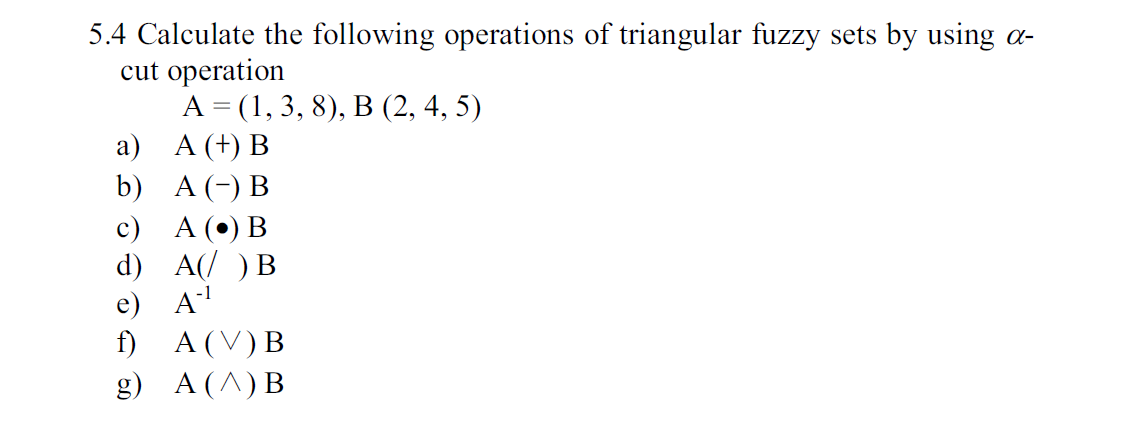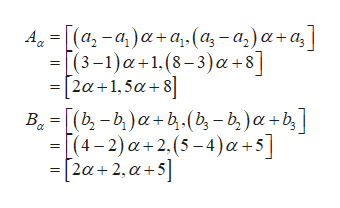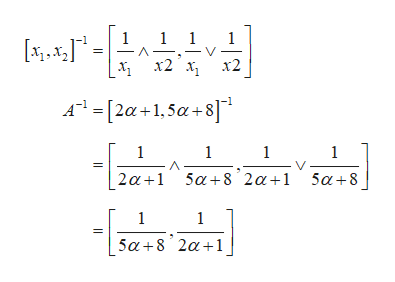5.4 Calculate the following operations of triangular fuzzycut operationА 3 (1, 3, 8), В (2, 4, 5)а) А (+) Вb) A Bsets by usingс) А () Вd) A Be) Af)A (V) BA (A) Bg)

Question

5.4 the book is "First Course on Fuzzy Theory and Applications"

please, I need the solution of part  e, f, and ghelp_outlineImage Transcriptionclose5.4 Calculate the following operations of triangular fuzzy cut operation А 3 (1, 3, 8), В (2, 4, 5) а) А (+) В b) A B sets by using с) А () В d) A B e) A f) A (V) B A (A) B g) fullscreen
Step 1

Consider the following given fuzzy sets:

Step 2

Now use the formulas:help_outlineImage TranscriptioncloseА, - [(а, -а,)а-а, (а, - а,)а-а,] - (3-1)а+1.(8-3)а -8] - [2а +1,5а + 8] В. - [(ъ, - b)а-b. (b, - b,)a+b,| - [(4-2) а+2.(5-4)a +5] - [2а + 2, а +5] fullscreen
Step 3

Now, consider (e) part and use...help_outlineImage Transcriptionclose1 1 ΕΓ. Η::1. A-[2α+1, 5α + 8]" 1 1 1 1 2α+15α + 8'2α+1 5α+8 1 1 5α+8'2α+1, fullscreen

Want to see the full answer?

See Solution

Want to see this answer and more?

Our solutions are written by experts, many with advanced degrees, and available 24/7

See Solution
Tagged in

Math